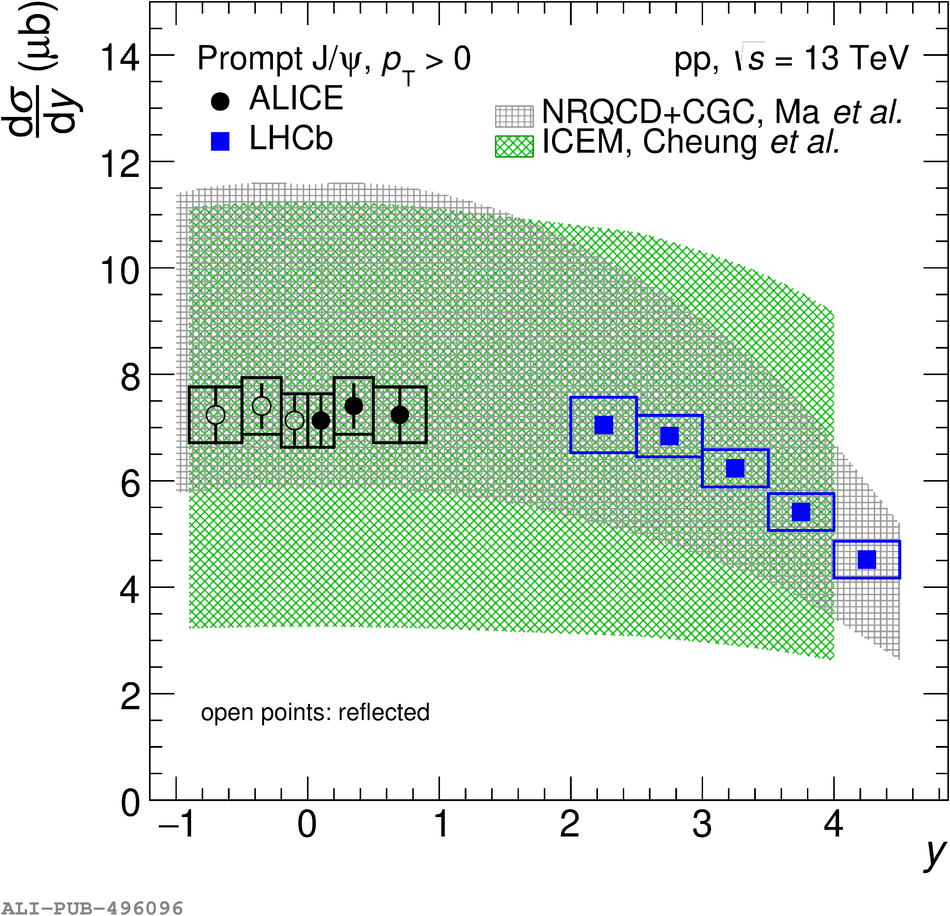# Figure 5

 Left panel: the $\frac{{\rm d}\sigma}{{\rm d}y}$ of non-prompt \pJPsi extrapolated down to \pt\ = 0 at midrapidity computed by the ALICE collaboration compared with similar measurements in pp collisions at \s = 13 TeV carried out at forward rapidity by the LHCb collaboration  Right panel: the $\frac{{\rm d}\sigma}{{\rm d}y}$ of prompt \pJPsi as a function of the rapidity in \pp collisions at \s = 13 TeV. The results close to midrapidity are based on the ALICE measurements extrapolated down to \pt = 0, and closed (open) symbols represent measured (reflected) data points (see text for details). Similar results obtained by the LHCb collaboration  at forward rapidity are shown as well. The theoretical calculations are from Refs. .